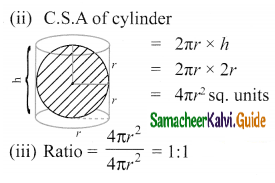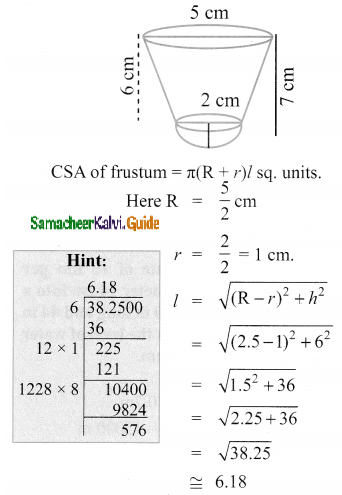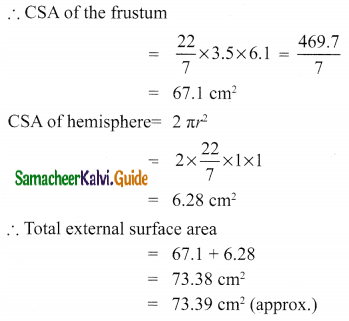Students can download Maths Chapter 7 Mensuration Ex 7.3 Questions and Answers, Notes, Samacheer Kalvi 10th Maths Guide Pdf helps you to revise the complete Tamilnadu State Board New Syllabus, helps students complete homework assignments and to score high marks in board exams.

## Tamilnadu Samacheer Kalvi 10th Maths Solutions Chapter 7 Mensuration Ex 7.3

Question 1.
A vessel is in the form of a hemispherical bowl mounted by a hollow cylinder. The diameter is 14 cm and the height of the vessel is 13 cm. Find the capacity of the vessel.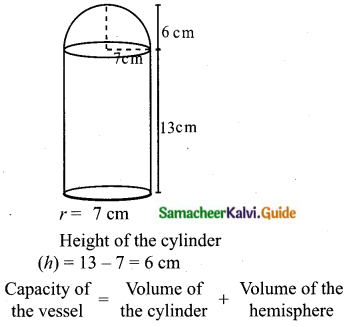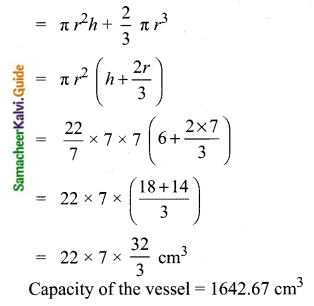Question 2.
Nathan, an engineering student was asked to make a model shaped like a cylinder with two cones attached at its two ends. The diameter of the model is 3 cm and its length is 12 cm. If each cone has a height of 2 cm, find the volume of the model that Nathan made.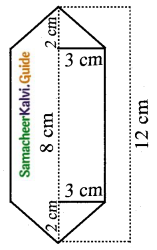r = $$\frac{3}{2}$$ cm
Height of the cone (H) = 2 cm
Height of the cylinder (h) = 12 – (2 + 2) cm = 8 cm
Volume of the model = Volume of the cylinder + Volume of 2 cones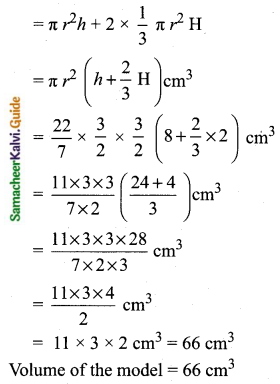Question 3.
From a solid cylinder whose height is 2.4 cm and the diameter 1.4 cm, a cone of the same height and same diameter is carved out. Find the volume of the remaining solid to the nearest cm3.
Radius of a cylinder = Radius of a cone r = 0.7 cm
Height of a cylinder = Height of a cone (h) = 2.4 cm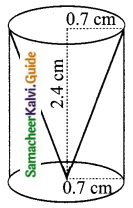Volume of the remaining solid = Volume of the cylinder – Volume of a cone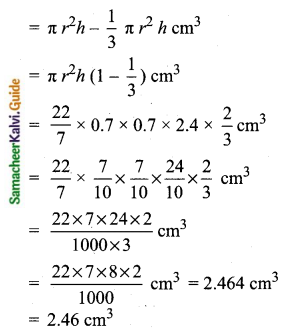Volume of the remaining soild = 2.46 cm3

Question 4.
A solid consisting of a right circular cone of height 12 cm and radius 6 cm standing on a hemisphere of radius 6 cm is placed upright in a right circular cylinder full of water such that it touches the bottom. Find the volume of the water displaced out of the cylinder, if the radius of the cylinder is 6 cm and height is 18 cm.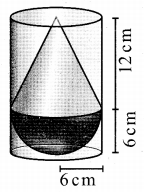r = 6 cm
Height of a cone (h) = 12 cm
Volume of the water displaced = Volume of the solid inside = Volume of the cone + Volume of the hemisphere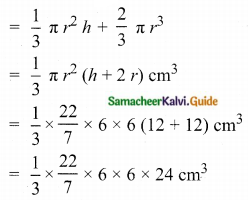Volume of water displaced = 905. 14 cm3.Question 5.
A capsule is in the shape of a cylinder with two hemispheres stuck to each of its ends. If the length of the entire capsule is 12 mm and the diameter of the capsule is 3 mm, how much medicine it can hold?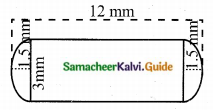r = $$\frac{3}{2}$$ mm = 1.5 mm
Height of the cylinderical portion = 12 mm – (1.5 mm + 1.5 mm) = (12 – 3) mm = 9 mm
Volume of the capsule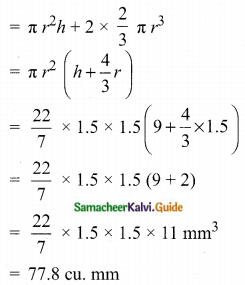Volume of the capsule = 77.8 cu. mm

Question 6.
As shown in figure a cubical block of side 7 cm is surmounted by a hemisphere. Find the surface area of the solid.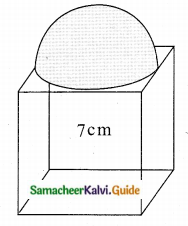Side of a cube (a) = 7 cm
Radius of a hemisphere (r) = $$\frac{7}{2}$$ cm
Surface area of the solid = T.S.A of the cube + C.S.A of the hemisphere – Area of the base of the hemisphere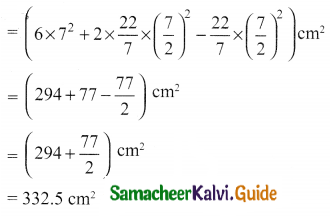Question 7.
A right circular cylinder just encloses a sphere of radius r units. Calculate
(i) the surface area of the sphere
(ii) the curved surface area of the cylinder
(iii) the ratio of the areas obtained in (i) and (ii).